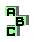CIRCUMCIRCLE    (5/13)

 r447 antimedial triangle, orthocenter, circumcenter, Brocard line r450 circumconic, circumcenter, orthocenter, isogonal points, isogonal of the infinite point r453 orthocenter, orthic triangle, incenter, homothetic triangles r469 Vecten points, Vecten squares r477 antipedal line, circumtangential triangle r478 ninecircle, Simson line, tangent circles r483 isogonal points, circumcenter, cevian triangle, orthopole r484 orthopole, inverse points r497 orthopole, isogonal points, inverse points r499 orthic triangle, homothetic triangles r500 isogonal points, point X(33), Soddy line, incenter r501 incenter, excenters, Nagel point r502 incenter, excenters, orthocenter, circumcenter, point X(84) r503 excenters, extouch triangle r507 point X(115), point X(110), Parry point, Parry reflection point, Parry circle, concyclic points r511 isogonal points, inverse points, similar triangles r520 isogonal of the infinite point, isogonal points, circumcevian triangle, circumconic r530 altitudes, orthocenter, antimedial triangle r534 pedal triangle, tangential triangle r540 antimedial triangle, cevian triangle r544 r557 medians, symmedian lines, Simson line r566 cevian triangle r568 Fermat triangles1 2 3 4 5 6 7 8 9 10 11 12 13